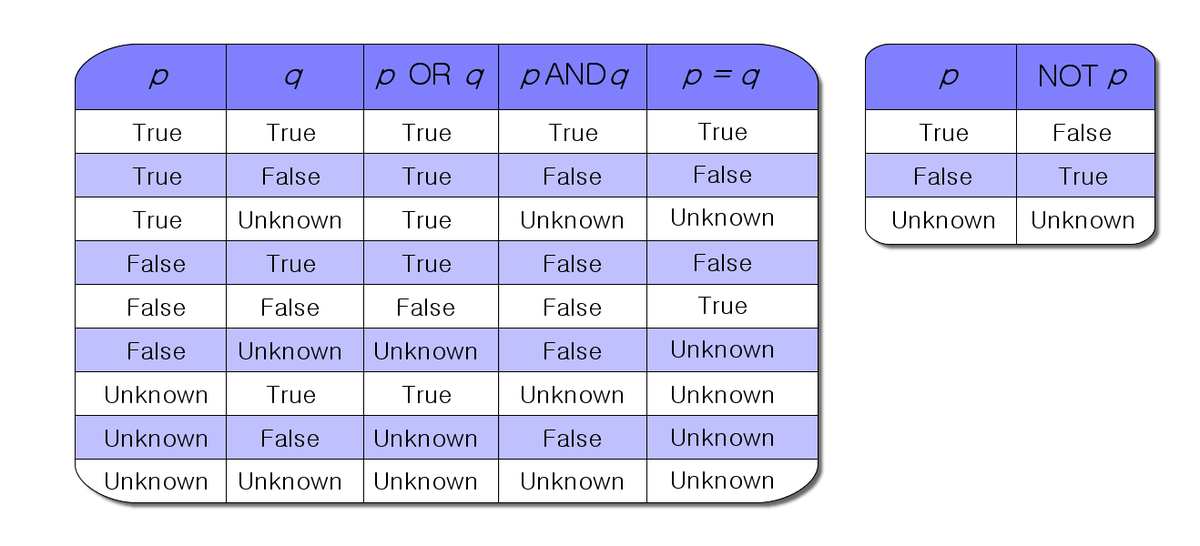# Propositional Rules, Logical Decision Trees, and the Satisfiability ProblemHere is a question I received the other day:

Can the propositional rules generated by logical decision trees be recast as a satisfiability problem, making decision trees NP-complete?

I’m going to break my answer down into segments.

# What are Propositional Rules?

In order to understand what proposition rules are, it is important to understand what a proposition is. A proposition is the fundamental building block of logic.

A proposition is a sentence that is either true or false, but not both. If a proposition is true, it is denoted by the capital letter T. If a proposition is false, it can be denoted by the capital letter F. Below are some examples of propositions:

• The sky is blue. …. T
• 10 + 10 = 20. ….T
• The letter “p” is a vowel…..F

Given two propositions p and q, we can create a “if p then q” statement that is known as an implication. Implications can be written as p → q. Here is an example of an implication:

• p = The relative humidity is greater than 70%
• q = It is raining tonight
• p → q = “If the relative humidity is greater than 70% then it is raining tonight.”

As seen in the real-world example above, propositional rules can be created by combining propositions in different ways.

# What is the Satisfiability Problem?

If I have a propositional formula denoted as a vector of boolean variables, for example x = (x1 = F, x2 = T, x3 = F, x4 = T, x5 = F), can we find an assignment of values for these boolean variables that would evaluate the propositional formula to True? This statement denotes that satisfiability problem.

Consider, for example. The following formula:

• F = x1 AND x2 AND x3 AND x4 AND x5

The formula denoted as F above will evaluate to TRUE if all the variables in the vector x are assigned true. Therefore, the formula is satisfiable.

This satisfiability problem was proven to be NP-complete by Stephen A. Cook.

# What does NP-Complete Mean?

Computational problems that belong to the class of NP-complete problems are those that have no known efficient (polynomial time) solution algorithms. You can beat your head against a wall trying to find a fast solution to these sorts of problems. However, given solution to these NP-complete problems, you can verify that solution in polynomial time (i.e. quickly).

An example of an NP-complete problem is the Vertex Cover Problem some of you might have seen in an algorithms or data structures class.

# Can the Propositional Rules Generated by Logical Decision Trees Be Recast as a Satisfiability Problem, Making Decision Trees NP-Complete?

Yes they can. Once we have the propositional rules, we then need to either find a variable assignment that makes it satisfiable, or we can report that no such assignment exists.

Let’s suppose we are dealing with binary decision trees, and we have three variables, a, b, and c. There are 2^n possible truth assignments that would need to be verified.

By the way, there is a famous paper by Hyafil and Rivest proving that optimal construction of decision trees is NP-complete.

# References

Cook, S. A. and Mitchell, D. G. “Finding Hard Instances of the Satisfiability Problem: A Survey.” In Satisfiability Problem: Theory and Applications (Piscataway, NJ, 1996) (Ed. D. Du, J. Gu, and P. M. Pardalos). Providence, RI: Amer. Math. Soc., pp. 1-17, 1997.